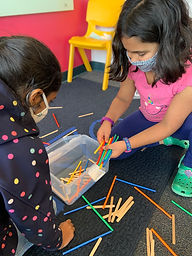## Mr. Rodney

### Target 1​

###### Lesson Type:

Review

Data Probability

:

Interpreting Data

Construct and use information presented in a table to solve problems.

###### 1:

Understand that data located in a table or grid is a combination of both row and column.

###### 2:

Understand that rows and columns in a table have headers that indicate the type of data.

3rd

###### Vocabulary:

Table, Data, Information

Activities:

• Took turns throwing straws and pipsicle sticks into a bin to determine which object made for a better "Cupid's Arrow."
• Accuracy was first compared based on limited quantities (5 throws per child) and then again with unlimited throws within a 15 second span.
• Students collected data in a table and recorded the outcomes of each round in fraction form and simplified (informally) where possible.### Home Exploration

###### Guiding Questions:## Absent Students:

### Target 2

:

###### 1:

Identify the correct arithmetic processes based on the information presented in word problems.

###### 2:

Find the key words in word problems that indicate the correct arithmetic process to use.

###### 3:

Find and use the needed information in a word problem in order to solve.

###### 4:

Write an equation from a word problem with a variable included in the equation. Then solve for the variable.

3rd

###### Vocabulary:

Word Problems, Equation, Information,

Activities:

• Solved word problems using data extracted from the table in the first activity, focusing on multiplication and division skills.
• Identified the relevant information to determine the operation needed to solve the problem.
• Wrote an equation to best represent the question being asked and solved.
• For problems that required larger numbers outside of students' comfort level, the emphasis was placed more on writing the equation than their ability to solve.
• Discussed the possibility of a word problem having irrelevant information, included to distract or confuse the reader.### Home Exploration

What is the imporant information we need from this problem? Lets underline or highlight it.

Where can we find the information that we need?

What operation will we need to use in our equation?

###### Guiding Questions:### Target 3

:

###### Vocabulary:

Activities:### Home Exploration